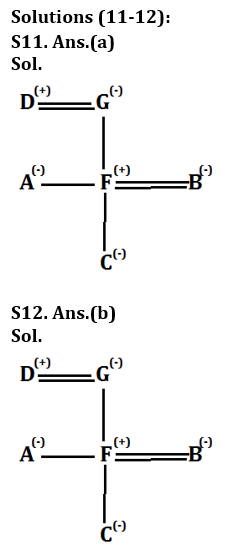Latest Banking jobs   »

# Reasoning Ability Quiz For IDBI AM/ Bank of India PO 2023 -8th March

Directions (1-5): Study the following arrangement carefully and answer the questions given below:
N 4 7 T U J K 3 % F @ © L N 5 P 1 8 U \$ E 2 D C 6 # 9 Z Q 8 Y*M A

Q1. Which of the following element is sixth to the left of the fifteenth from the left end of the given arrangement?
(a) 2
(b) #
(c) %
(d) \$
(e) None of these

Q2. How many such numbers are there in the given arrangement each of which is immediately preceded by a consonant but not immediately followed by a letter?
(a) One
(b) None
(c) Three
(d) Two
(e) Four

Q3. How many such symbols are there in the arrangement, each of which is immediately followed by a letter but not immediately preceded by a number?
(a) One
(b) Three
(c) None
(d) Two
(e) More than three

Q4. If we remove all the numbers from the given arrangement, then which of the following element is 5th from the right end?
(a) Q
(b) T
(c) *
(d) #
(e) None of these

Q5. Four of the following five are alike in a certain way based on their positions in the given arrangement and so from a group. Which is the one that does not belong to that group?
(a) DC6
(c) 9ZQ
(d) 5P1
(e) #MP

Directions (6-10): Study the following arrangement carefully and answer the questions given below:
3 1 2 5 4 7 2 8 4 5 7 1 9 6 5 2 4 1 4 5 8 2 4 3 9 7 6 9 4 2 3

Q6. Which of the following digit will be sixth to the left of tenth from the right end?
(a) 6
(b) 9
(c) 5
(d) 2
(e) None of these

Q7. How many 2’s is there in the given series which are followed by a number less than 6?
(a) One
(b) None
(c) Three
(d) Two
(e) More than three

Q8. What will be the addition of fifth, seventh and tenth digit from the left end?
(a) 13
(b) 10
(c) 11
(d) 15
(e) None of these

Q9. If all the perfect square digits are removed from the given series, then which of the following will be fifth to the right of sixth from the left end?
(a) 5
(b) 2
(c) 8
(d) 1
(e) None of these

Q10. How many odd numbers are there in the given series which are immediately followed by a perfect square digit?
(a) One
(b) None
(c) Three
(d) Five
(e) Seven

Direction (11-12): Study the following information carefully and answer the given questions.
There are six members in three generation family i.e. A, F, D, B, C, and G. Among them two married couple and only two males. G is mother-in-law of B. A who is unmarried, and F are siblings. B’s husband has only one child. D is father of F.

Q11. How is F related to C’s grandmother?
(a) Son
(b) Father
(c) Mother
(d) Daughter
(e) None of these

Q12. Who is daughter-in-law of A’s father?
(a) A
(b) B
(c) F
(d) Cannot be determined
(e) None of these

Q13. Pointing to a lady, Sumit says to his only son, “She is daughter in law of my mother’s daughter in law”. Sumit is the only Child of his parents. How is the lady related to Sumit?
(a) Sister
(b) Brother
(c) Daughter-in-law
(d) Wife
(e) Daughter

Q14. Showing a man on the stage, Lady said, “He is the only child of the person who is grandfather of my son. How is the man on stage related to lady?
(a) Husband
(b) Brother-in-law
(c) Brother
(d) Sister
(e) Nephew

Q15. A man says to a lady, “Your mother is the mother-in-law of my mother’s only child.” How is the lady related to the man?
(a) Sister-in-law
(b) Aunt
(c) Daughter
(d) Wife
(e) Granddaughter

Solutions

Solutions (1-5):
S1. Ans.(c)

S2. Ans.(e)
Sol. N47, K3%, C6#, P18

S3. Ans.(b)

S4. Ans.(a)

S5. Ans.(e)

Solutions (6-10):
S6. Ans. (d)
Sol. The 16th element from the right end = ‘2’.

S7. Ans. (e)
Sol. There are four 2’s which are followed by number less than 6 i.e. ‘2 5, 2 4, 2 4 and 2 3’.

S8. Ans. (c)
Sol. The fifth, seventh and tenth digit from the left end are ‘4, 2 and 5′.
Hence, the sum is = (4+2+5) = 11.

S9. Ans. (b)
Sol. After removing all the perfect square digits ‘3 2 5 7 2 8 5 7 6 5 2 5 8 2 3 7 6 2 3’
Then (6+5) = 11th digit from the left is ‘2’.

S10. Ans. (e)
Sol. There are seven odd digits which are followed by a perfect square number- ‘3 1, 5 4, 7 1, 1 9, 1 4, 3 9, 9 4’’S13. Ans. (c)

S14. Ans. (a)

S15. Ans. (d)## FAQs

### What is the selection process of the IDBI AM?

The selection process of IDBI AM is Online exam and Interview.

#### Congratulations!Union Budget 2023-24: Free PDF